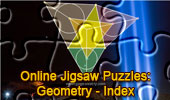# Jigsaw Puzzles: Geometry for Children

 Jigsaw Puzzles Geometry IndexJigsaw Puzzle: Pascal's Theorem.24 Pieces. Geometry: Jigsaw Puzzle: Marion Walter's Theorem.32 Quadrilateral Pieces. Puzzle: Exeter PointTriangle, Median, Circumcircle, Concurrent Lines, Euler Line. Clawson Point Puzzle, Geometry for Kids. 22 Piece Polygons HTML5 canvas for iPad, Nexus and touch Devices. Jigsaw Puzzle Ptolemy's Theorem. 22 Piece Polygons. Jigsaw Puzzle: Problem 1182: Two Squares. 35 Pieces. Jigsaw Puzzle Geometry Problems 221 - 230. 22 Piece Polygons. Jigsaw Puzzle: Geometry Problems 161 - 170. 22 piece polygons. Area, Triangle, Parallelogram, Trapezoid, Pentagon, Diagonal, Midpoint, Octagon. Jigsaw Puzzle based on the Kosnita's Theorem Kosnita's Theorem. Circumcenters, Concurrent lines. Jigsaw Puzzle based on the problem 410: 22 piece polygons. Two Regular Pentagons, Angle. . Jigsaw Puzzle based on problem 401. Area, Triangle, Angle bisector, Circumcircle, Perpendicular bisector, Congruence. Jigsaw Puzzle based on the problem 368. Triangle, 120 degrees, Angle bisectors, Perpendicular. . Puzzle: Euclid's Elements Book I, 23 Definitions. One-page visual illustration. Puzzle: Marion Walter's theorem. Puzzle cut: 12 Piece ZigZag Based on Marion Walter's theorem Intouch and Extouch Triangles. Puzzle cut: 20 Piece Classic Based on Proposed Problem 86. Contact Triangles. Puzzle cut: 22 Piece Polygons Based on Proposed Problem 85. Pentagons & Pentagrams - Puzzle: 96 triangles. Puzzle of the Newton's Theorem: 50 pieces of circles. Euler's Formula - Puzzle: 55 pieces in a six star style of piece. Puzzle of the Nagel Point: 22 pieces of polygons. Equal Incircles Theorem: 20 Piece Classic. Puzzle of the Ceva's Theorem: 48 classic piece. Morley's Theorem Puzzle: 22 pieces of polygons.